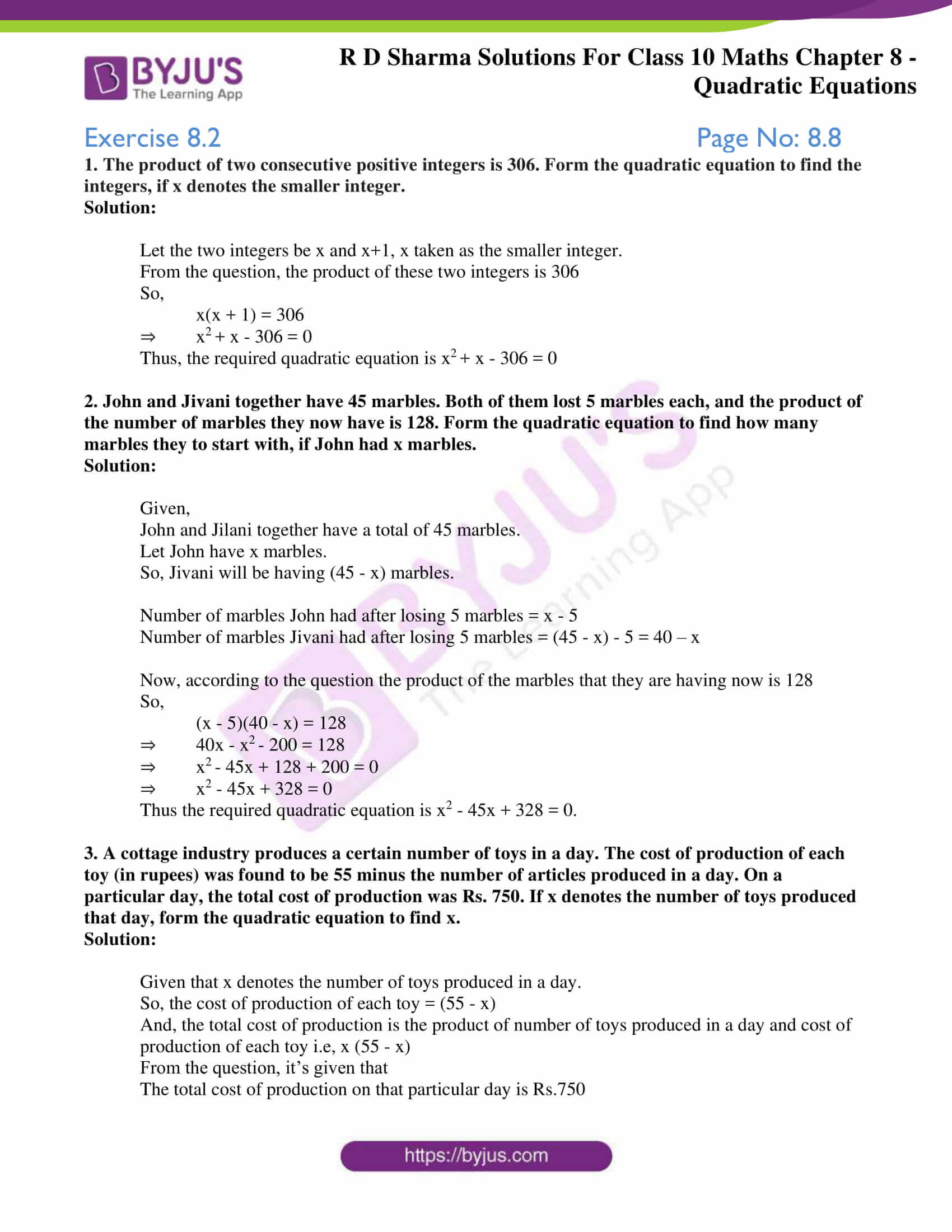# RD Sharma Solutions for Class 10 Maths Chapter 8 Quadratic Equations Exercise 8.2

Quadratic equations arise in many situations around us and in different fields of mathematics. The following exercise helps students formulate the given problem into a quadratic equation. For a clear understanding of how to solve these problems, students can refer the RD Sharma Solutions Class 10 sincerely prepared by field experts at BYJU’S in a simple language. The PDF containing solutions of RD Sharma Solutions for Class 10 Maths Chapter 8 Quadratic Equations Exercise 8.2 is given below.### Access RD Sharma Solutions for Class 10 Maths Chapter 8 Quadratic Equations Exercise 8.2

1. The product of two consecutive positive integers is 306. Form the quadratic equation to find the integers, if x denotes the smaller integer.

Solution:

Let the two integers be x and x+1, x taken as the smaller integer.

From the question, the product of these two integers is 306

So,

x(x + 1) = 306

⇒ x+ x – 306 = 0

Thus, the required quadratic equation is x+ x – 306 = 0

2. John and Jivani together have 45 marbles. Both of them lost 5 marbles each, and the product of the number of marbles they now have is 128. Form the quadratic equation to find how many marbles they to start with, if John had x marbles.

Solution:

Given,

John and Jilani together have a total of 45 marbles.

Let John have x marbles.

So, Jivani will be having (45 – x) marbles.

Number of marbles John had after losing 5 marbles = x – 5

Number of marbles Jivani had after losing 5 marbles = (45 – x) – 5 = 40 – x

Now, according to the question the product of the marbles that they are having now is 128

So,

(x – 5)(40 – x) = 128

⇒ 40x – x– 200 = 128

⇒ x2 – 45x + 128 + 200 = 0

⇒ x2 – 45x + 328 = 0

Thus the required quadratic equation is x2 – 45x + 328 = 0.

3. A cottage industry produces a certain number of toys in a day. The cost of production of each toy (in rupees) was found to be 55 minus the number of articles produced in a day. On a particular day, the total cost of production was Rs. 750. If x denotes the number of toys produced that day, form the quadratic equation to find x.

Solution:

Given that x denotes the number of toys produced in a day.

So, the cost of production of each toy = (55 – x)

And, the total cost of production is the product of number of toys produced in a day and cost of production of each toy i.e, x (55 – x)

From the question, it’s given that

The total cost of production on that particular day is Rs.750

So,

⇒ x (55 – x) = 750

⇒ 55x – x2 = 750

⇒ x– 55x + 750 = 0

Thus, the required quadratic equation is x– 55x + 750 = 0.